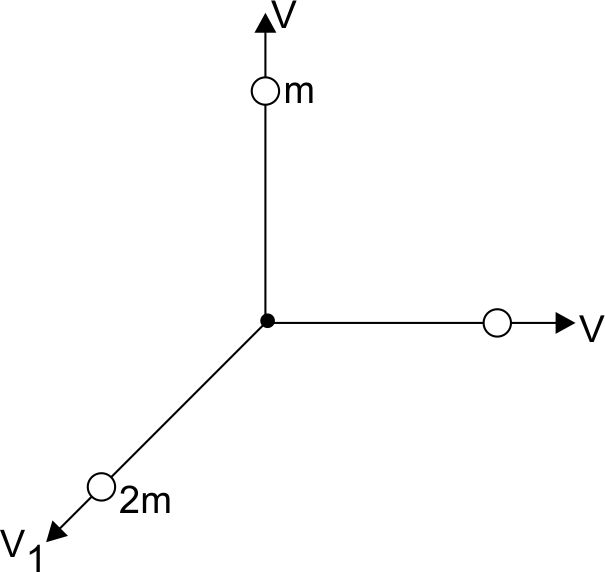#### A body of mass (4m) is lying in x-y plane at rest. It suddenly explodes into three pieces. Two pieces, each of mass (m) move perpendicular to each other with equal speeds (V). The total Kinetic energy generated due to explosion is Option 1)Option 2)Option 3)Option 4)As we learnt in

Conservation of Energy and Conservation of Mechanical Energy -

Accounting all forms of energy within isolated system

i.e. total energy remains constant.

-Total K.t. =Option 1)This solution is incorrect

Option 2)This solution is correct

Option 3)This solution is incorrect

Option 4)This solution is incorrect

## Crack NEET with "AI Coach"

• HD Video Lectures
• Unlimited Mock Tests
• Faculty Supportseems logical .we assume that the 3rd part ie mass 2m goes in (-x-y) plane though the problem does not specify.How to explain that mass 2m does not move in Z direction?